• Slides: 32Adding Numbers + + H T U 2 4 6 6 3 2 4 2 0 1 2 7 5 6 2 3 8 3 7 3 6 8 8 6 5 8 H T U 5 5 9 3 4 6 7 4 1 + 9 0 2 5 2 3 7 0 0 1 2 4 8 5 9 7 www. visuallessons. com + + +Adding Decimals T U 1/10 3 0 7 2 4 9 3 7 3 4 2 9 7 2 7 1 1 7 7 3 6 9 7 6 4 9 0 T U 1/10 3 0 9 5 2 9 6 7 2 7 + 7 0 2 3 0 9 2 5 3 3 7 9 7 6 0 5 9 8 0 + www. visuallessons. com + +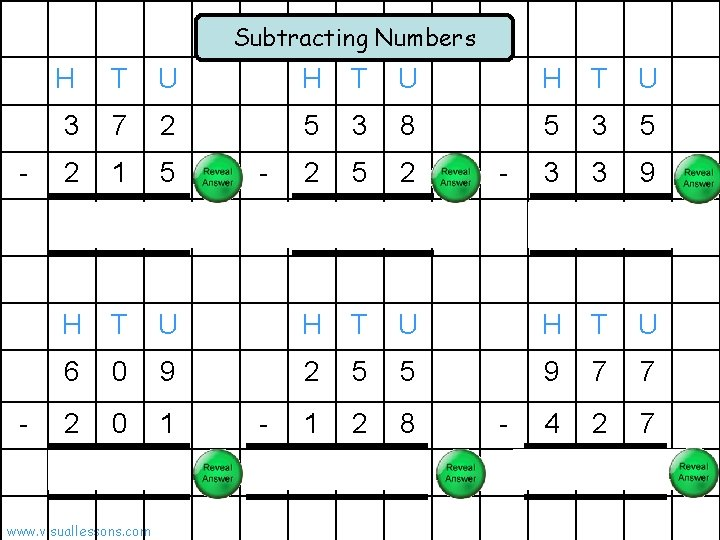Subtracting Numbers - - H T U 3 7 2 5 3 8 5 3 5 2 1 5 2 3 3 9 1 5 7 2 8 6 1 9 6 H T U 6 0 9 2 5 5 9 7 7 2 0 1 1 2 8 4 2 7 4 0 8 1 2 7 5 5 0 www. visuallessons. com - -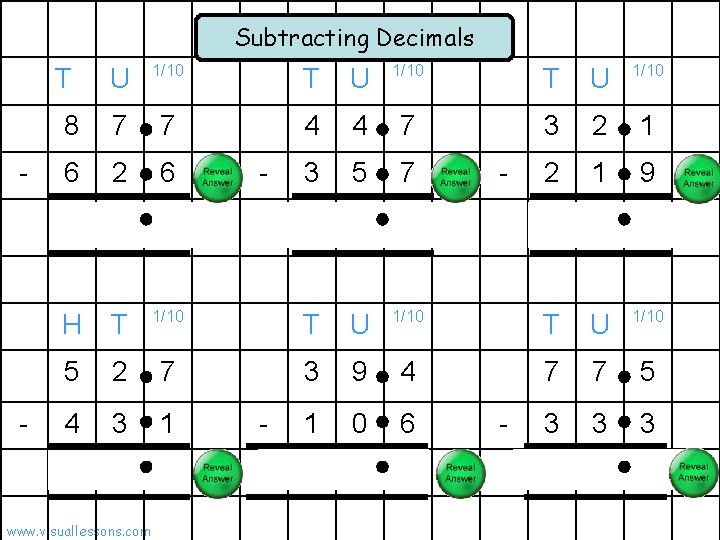Subtracting Decimals - - T U 1/10 8 7 7 4 4 7 3 2 1 6 2 6 3 5 7 2 1 9 2 5 1 9 0 1 0 2 H T 1/10 T U 1/10 5 2 7 3 9 4 7 7 5 4 3 1 1 0 6 3 3 3 9 6 2 8 8 4 4 2 www. visuallessons. com - -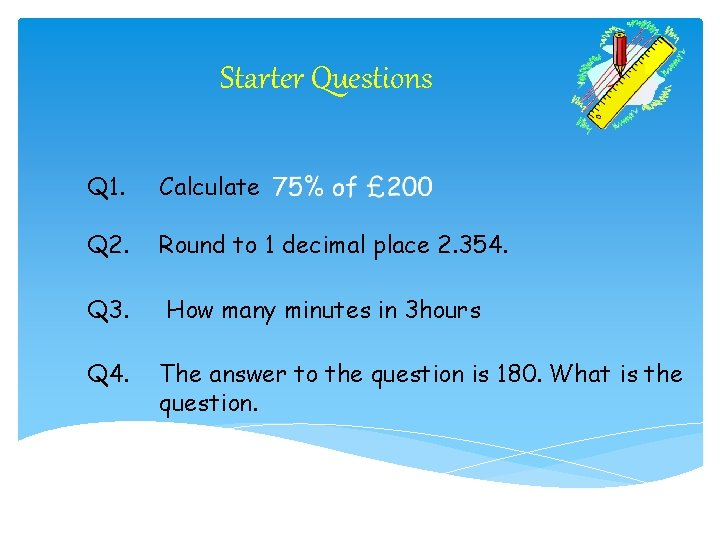Starter Questions Q 1. Calculate Q 2. Round to 1 decimal place 2. 354. Q 3. How many minutes in 3 hours Q 4. The answer to the question is 180. What is the question.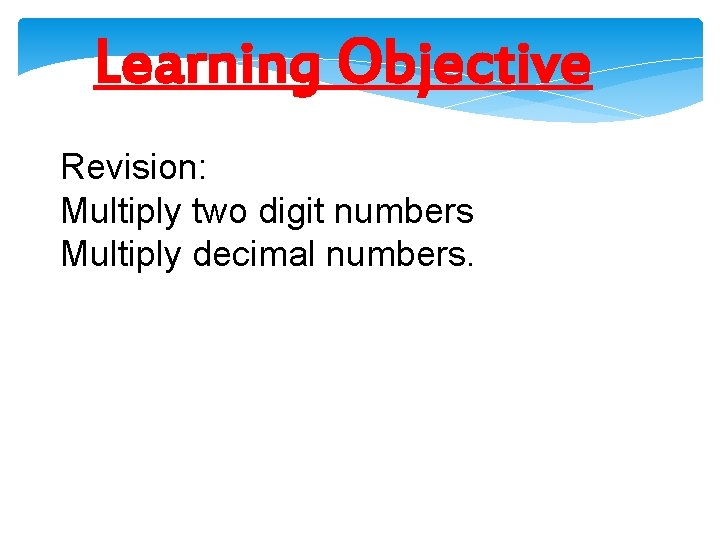Learning Objective Revision: Multiply two digit numbers Multiply decimal numbers.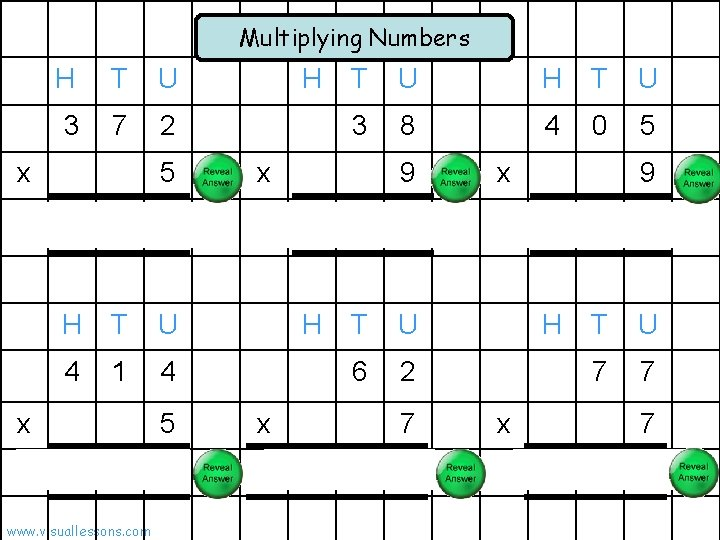Multiplying Numbers H T U 3 7 2 x 1 5 T U H T U 3 8 4 0 5 x 9 x 3 8 6 0 3 4 2 H T U 4 1 4 6 2 x 2 H 5 0 7 www. visuallessons. com 0 x 7 4 3 4 9 6 4 5 H T U 7 7 x 7 5 3 9Multiplying 2 Digit Numbers: Grid Method 3 x 5 x 3 30 5 30 900 150 1050 4 120 20 1 1 9 www. visuallessons. com 4 6 1 x 2 60 1 20 1200 20 1220 +140 3 180 3 +183 0 = 1 4 0 3 3Multiplying 2 Digit Numbers: Grid Method 2 x 3 x 6 20 3 60 1200 180 1380 6 120 18 1 5 1 www. visuallessons. com 6 3 5 x 2 30 5 20 600 100 700 +138 9 270 45 +315 8 = 1 0 1 9 5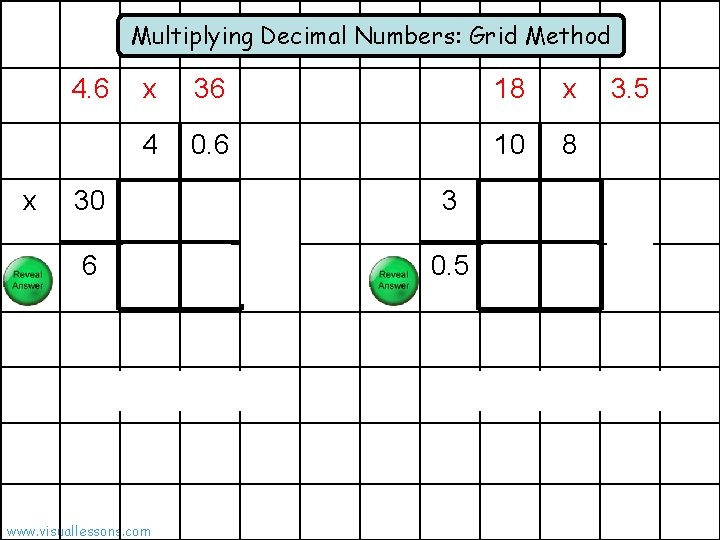Multiplying Decimal Numbers: Grid Method 4. 6 x x 36 18 x 4 0. 6 10 8 30 1200 180 1380 3 600 100 700 6 120 18 +138 0. 5 270 45 +315 1 8 = 1 0 1 www. visuallessons. com 3. 5 5Starter Questions MNU 2 -10 a MNU 2 -10 b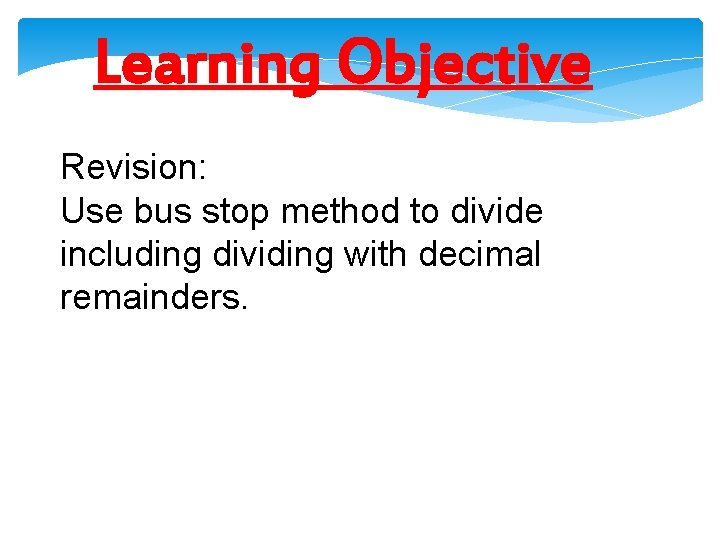Learning Objective Revision: Use bus stop method to divide including dividing with decimal remainders.Dividing Numbers 4 4 3 4 ÷ 7 6 2 6 1 5 ÷ 1 2 3 7 4 3 4 5 6 1 5 2 6 ÷ 3 6 5 4 ÷ 1 4 2 1 0 9 4 2 6 6 5 6 3 www. visuallessons. com 6 5 6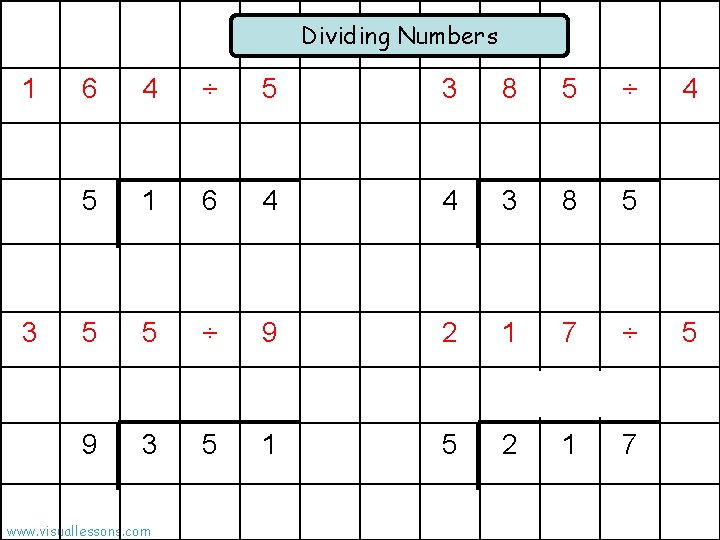Dividing Numbers 1 3 6 4 ÷ 5 3 8 5 ÷ 5 1 6 4 4 3 8 5 5 5 ÷ 9 2 1 7 ÷ 3 1 1 7 9 3 www. visuallessons. com 5 1 5 2 4 5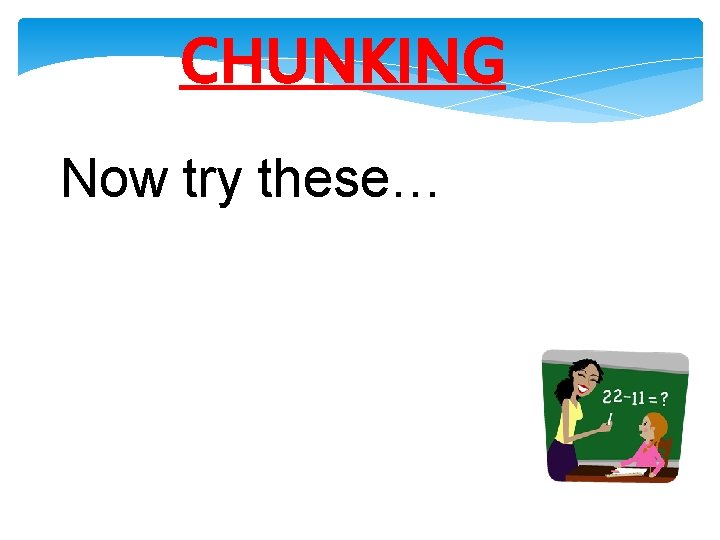CHUNKING Now try these…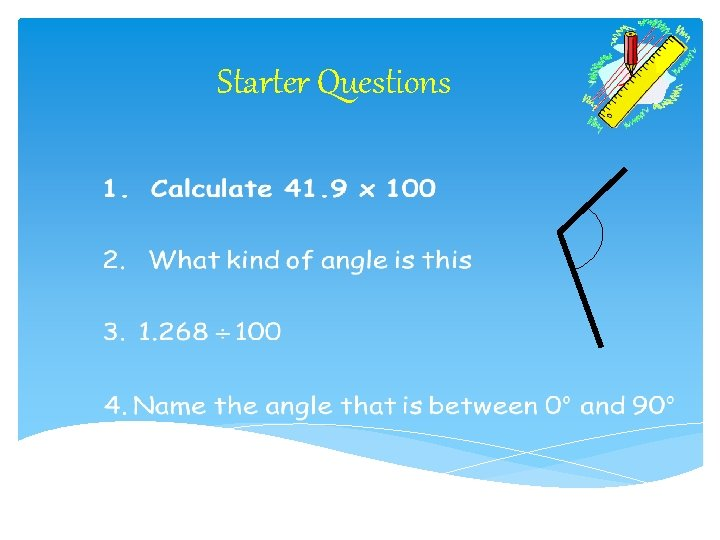Starter Questions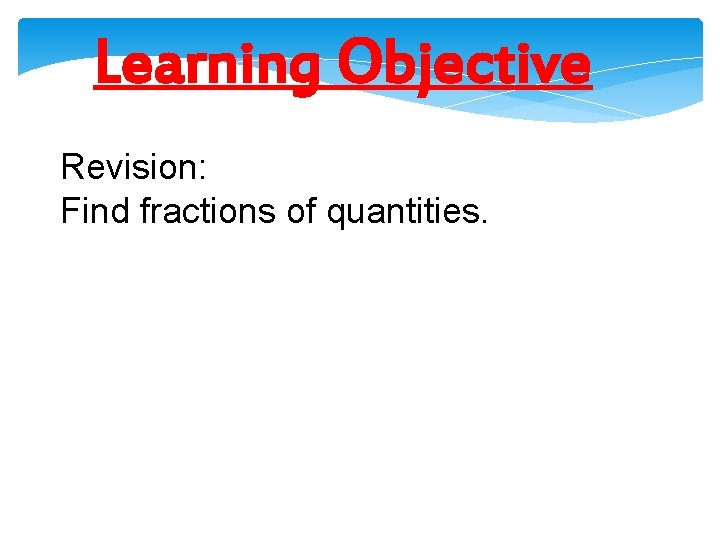Learning Objective Revision: Find fractions of quantities.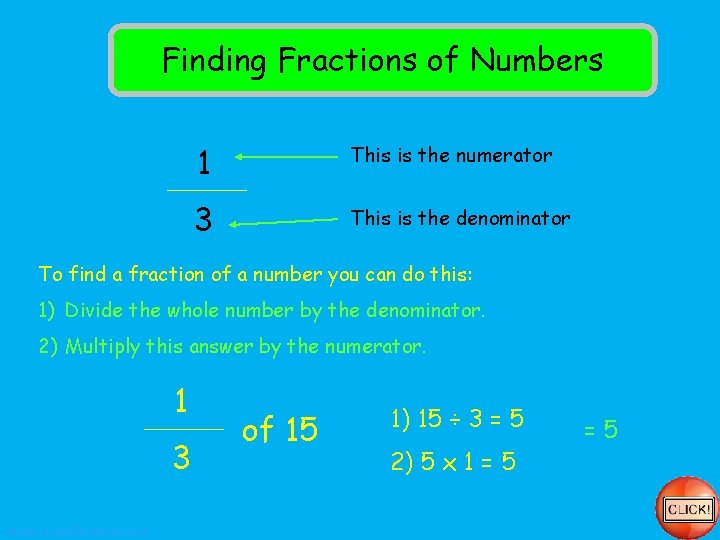Finding Fractions of Numbers 1 This is the numerator 3 This is the denominator To find a fraction of a number you can do this: 1) Divide the whole number by the denominator. 2) Multiply this answer by the numerator. 1 3 www. visuallessons. com of 15 1) 15 ÷ 3 = 5 2) 5 x 1 = 5 =5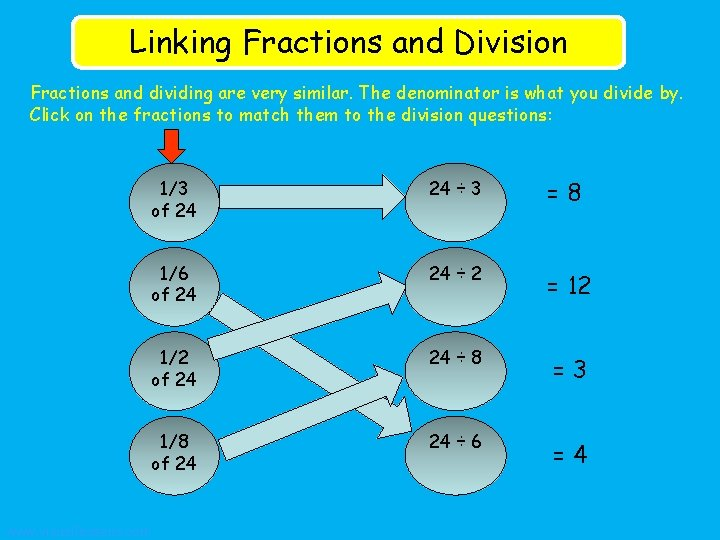Linking Fractions and Division Fractions and dividing are very similar. The denominator is what you divide by. Click on the fractions to match them to the division questions: www. visuallessons. com 1/3 of 24 24 ÷ 3 =8 1/6 of 24 24 ÷ 2 = 12 1/2 of 24 24 ÷ 8 =3 1/8 of 24 24 ÷ 6 =4Finding More Fractions of Numbers Divide the whole number by the denominator Multiply this answer by the numerator. Answer 2/3 of 21 21 ÷ 3 = 7 7 x 2 = 14 14 3/5 of 20 20 ÷ 5 = 4 4 x 3 = 12 12 3/4 of 16 16 ÷ 4 = 4 4 x 3 = 12 12 Remember: ü Divide the whole number by the denominator. ü Multiply this answer by the numerator. www. visuallessons. com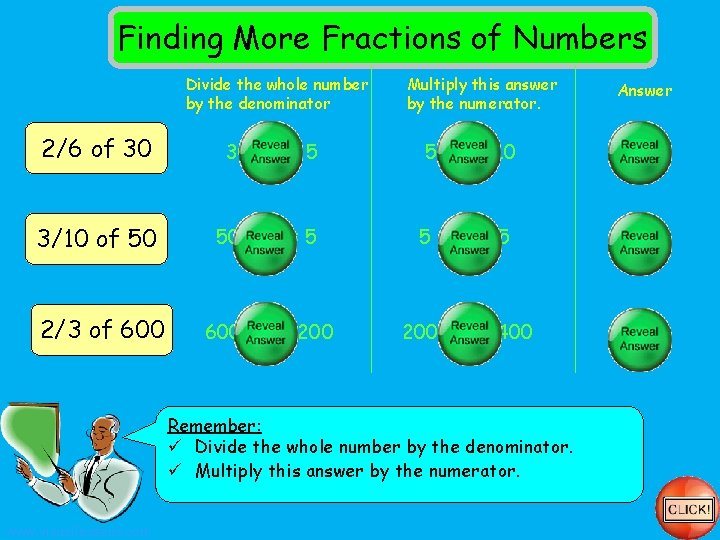Finding More Fractions of Numbers Divide the whole number by the denominator Multiply this answer by the numerator. Answer 2/6 of 30 30 ÷ 6 = 5 5 x 2 = 10 10 3/10 of 50 50 ÷ 10 = 5 5 x 3 = 15 15 2/3 of 600 ÷ 3 = 200 x 2 = 400 Remember: ü Divide the whole number by the denominator. ü Multiply this answer by the numerator. www. visuallessons. comFinding Fractions of Numbers Click on the question to reveal the answer 3040 ¾ of ¼ of 20 80 3/2012 of 80 7/1021 of 30 2/510 of 25 5/910 of 18 1/3 5 of 15 4/636 of 54 9 24 3/8 of 40 of 50 4/5 8 28 2/7 of 5/6 35 of 42 www. visuallessons. comUsing a Calculator to Find Fractions of Numbers Click on the question to reveal the answer ¾ 37. 5 of 50 ¼ of 90 22. 5 3/20 of 54 360 2/5126 of 315 6/7126 of 147 5/9195 of 351 1/3 63 of 189 2/6182 of 546 57152 3/8 of www. visuallessons. comStarter Questions Q 1. Convert to 24 hr clock 8. 15 am Q 2. Round to 1 decimal place 103. 525 Q 3. How many minutes in 6 hours Q 4. Find the time difference between 5. 12 am and 2. 34 pm 10. 02 pm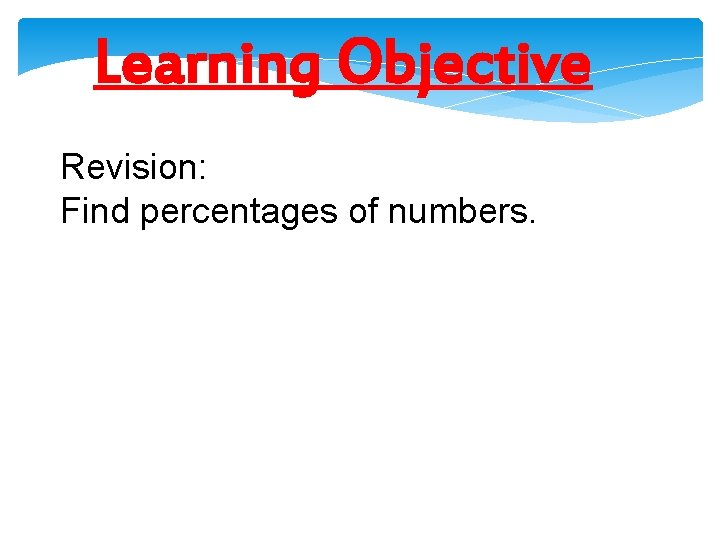Learning Objective Revision: Find percentages of numbers.Finding Percentages of Numbers 30% of 40 1) Find 10% first. This can be done by dividing the number by 10 10% of 40 = 4 2) If 10% = 4, then 30% = 12 (there are 3 lots of 10% in 30%) 30% of 40 = 12 www. visuallessons. com This box represents 40 100% = 40 90% = 36 80% = 32 70% = 28 60% = 24 50% = 20 40% = 16 30% =12 20% = 8 10% = 4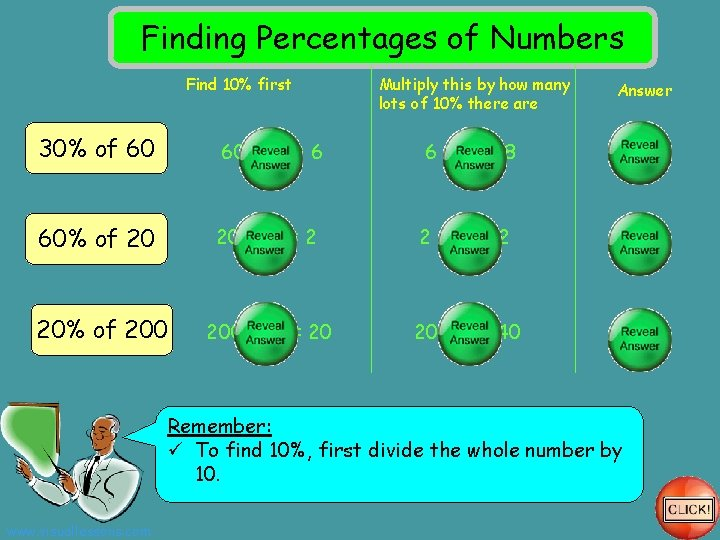Finding Percentages of Numbers Find 10% first Multiply this by how many lots of 10% there are Answer 30% of 60 60 ÷ 10 = 6 6 x 3 = 18 18 60% of 20 20 ÷ 10 = 2 2 x 6 = 12 12 20% of 200 ÷ 10 = 20 20 x 2 = 40 40 Remember: ü To find 10%, first divide the whole number by 10. www. visuallessons. com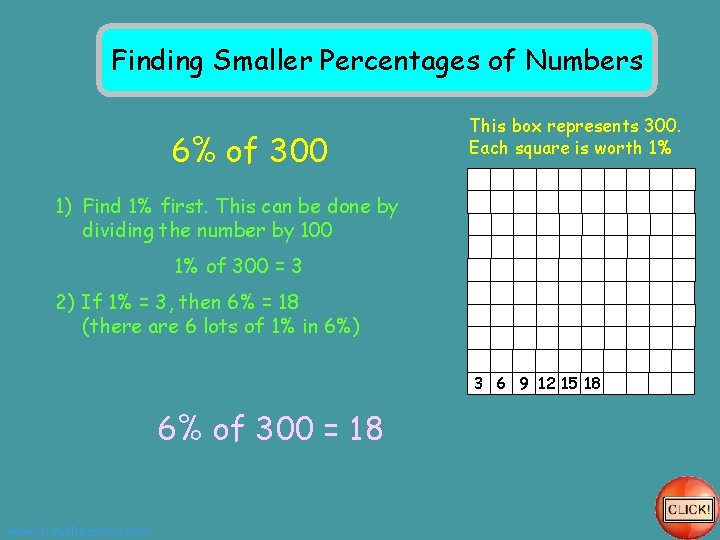Finding Smaller Percentages of Numbers 6% of 300 This box represents 300. Each square is worth 1% 1) Find 1% first. This can be done by dividing the number by 100 1% of 300 = 3 2) If 1% = 3, then 6% = 18 (there are 6 lots of 1% in 6%) 3 6 9 12 15 18 6% of 300 = 18 www. visuallessons. comFinding Percentages of Numbers Find 1% first Multiply this by how many lots of 1% there are Answer 6% of 400 ÷ 100 = 4 4 x 6 = 24 24 15% of 80 80 ÷ 100 = 0. 8 x 15 = 12 12 7% of 500 ÷ 100 = 5 5 x 7 = 35 35 Remember: ü To find 1%, first divide the whole number by 100. www. visuallessons. com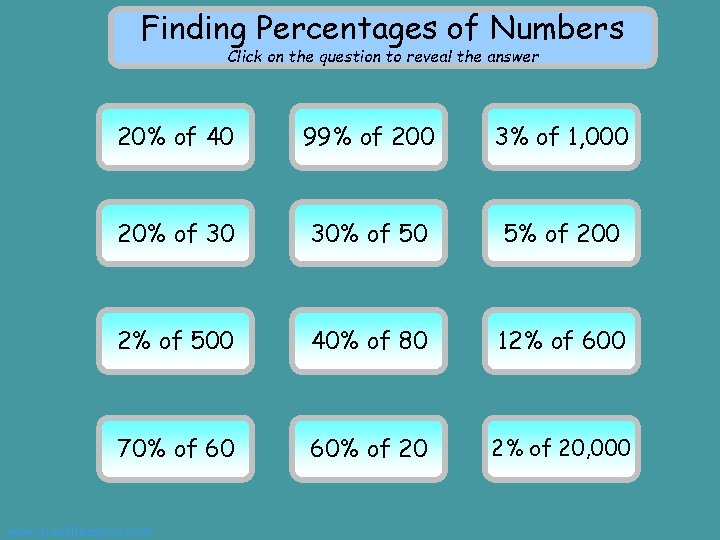Finding Percentages of Numbers Click on the question to reveal the answer 8 40 20% of 99% 198 of 200 3% of 30 1, 000 20% 6 of 30 30%15 of 50 10200 5% of 10500 2% of 32 of 80 40% 72600 12% of 70%42 of 60 60%12 of 20 2% of 400 20, 000 www. visuallessons. com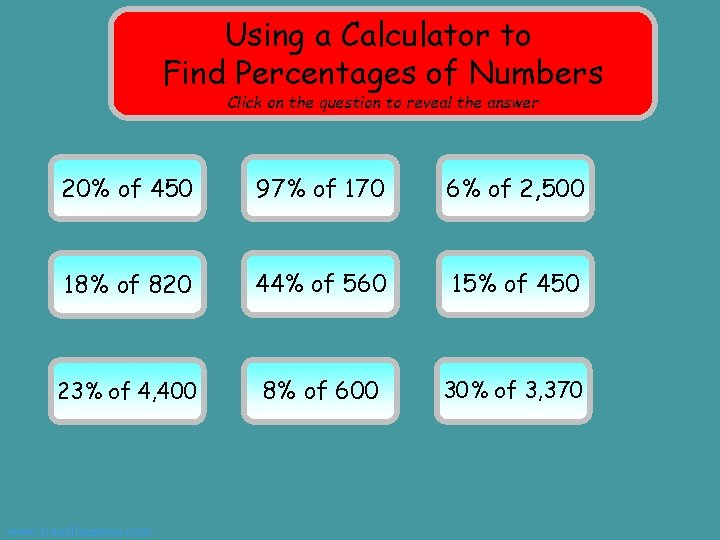Using a Calculator to Find Percentages of Numbers Click on the question to reveal the answer 90 450 20% of 97%164. 9 of 170 150 6% of 2, 500 18%147. 6 of 820 44% of 560 246. 4 15%67. 5 of 450 23% 1, 012 of 4, 400 8%48 of 600 30% 1, 011 of 3, 370 www. visuallessons. com